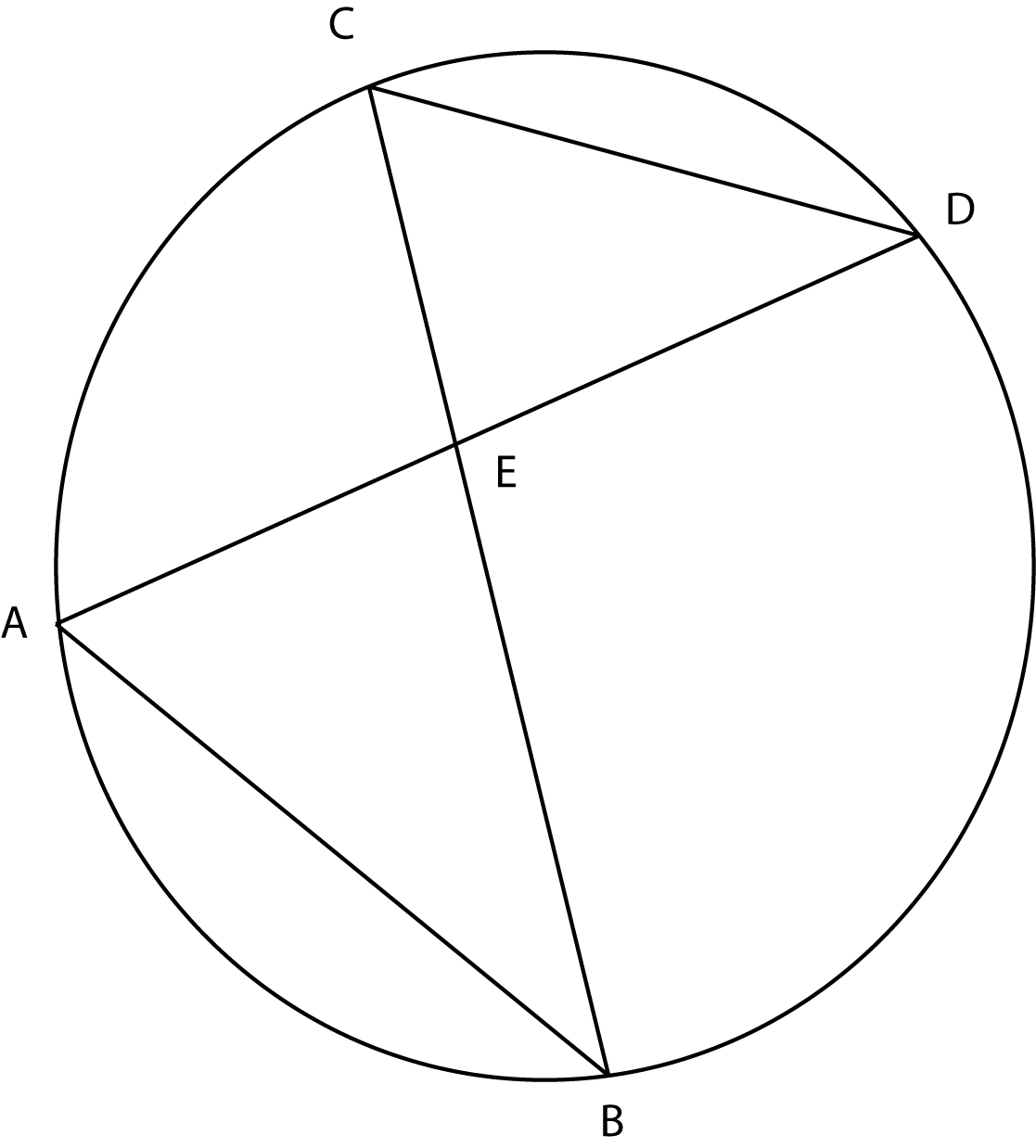### Prove: AE / CE = AB / CDClick or touch to draw on the diagram
Definition
∠DAB =∠DCB
Substitution
AA similarity
Inscribed angle measures half its intercepted arc
∠AEB = ∠CED
△AEB ~ △CED
∠DAB=$$\frac{1}{2}\overset{\large\frown}{DB}$$ and ∠DCB=$$\frac{1}{2} \overset{\large\frown}{DB}$$
AE / CE = AB / CD
Vertical angles are equal